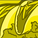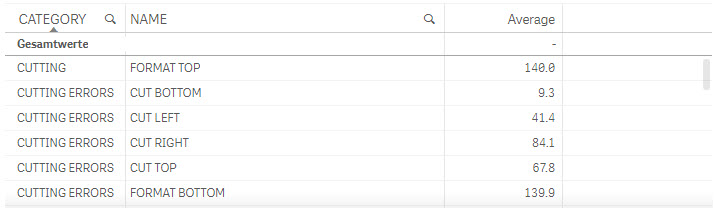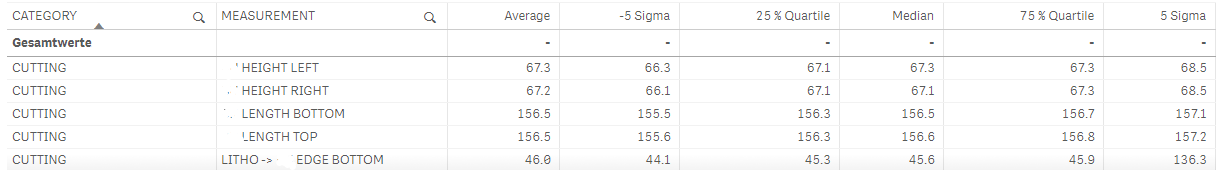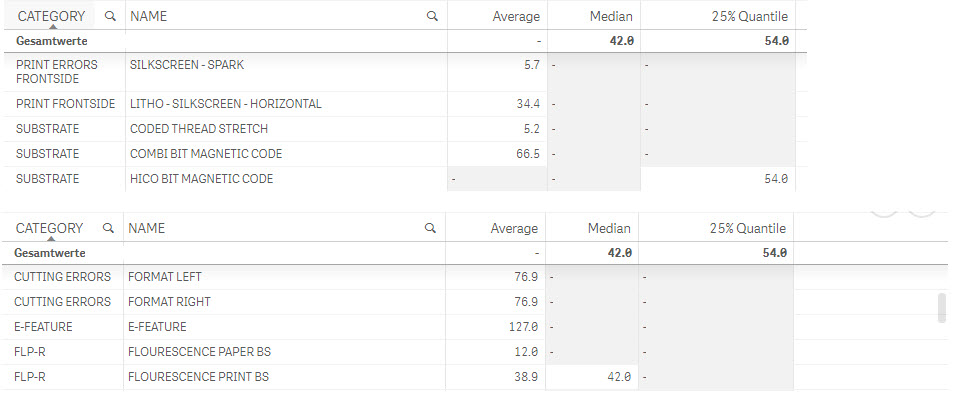# New to Qlik Sense

If you’re new to Qlik Sense, start with this Discussion Board and get up-to-speed quickly.

Announcements
Qlik Insider: SaaS capabilities for Data Integration and Analytics, May 25th! Register Now
cancel
Showing results for
Did you mean:Creator

## Weighted meridian in a straight table

Hi experts,

I try to apply a formula for calculating the median of weighted values (like in the post from Matt King Weighted median) in a straight table and I want to apply the formula from Marco Wedel to all dimension values in the table. I was able to calculate it for the average, because there you don't Need to Aggregate. Now I want to calculate the median for FORMAT TOP, CUT BOTTOM, ....

If I select a mesurement (NAME), then the calculation is working, but if I don't have anything selected, there is only 1 result for the whole table instead of one result per row.

I thought something like

Aggr({<COLLECTION={'SENSOR MEASUREMENTS'},RESULT_TYPE={'number'}>}

If(

((RangeSum(Above(Sum(HAEUFIGKEIT),0,RowNo())) /

Sum(TOTAL HAEUFIGKEIT))>=0.5) And

((RangeSum(Above(Sum(HAEUFIGKEIT),1,RowNo()-1)) /

Sum(TOTAL HAEUFIGKEIT))<0.5),

ERGEBNIS

),

(ERGEBNIS,(NUMERIC,ASCENDING)),NAME

)

might do the trick. But it doesn't and  I would be happy, if I got some hints about how to handle this.

Best regards

Dirk1 Solution

Accepted SolutionsCreator
Author

Hello everybody,

finally I found the solution for my Problem, based upon the design blog of HIC (thank you very much for this valuable explanation).

If somebody is looking for calculating the median or quantiles based upon pre-aggregated values and displaying the results in a straigt table (so you can have an overview of the results for different measurements), the following formula works for me.

Median:

Aggr({<RESULT_TYPE={'UNSIGNED_INT_7_BIT','SIGNED_INT_13_BIT'}>} NoDistinct

Only(If(Aggr({<RESULT_TYPE={'UNSIGNED_INT_7_BIT','SIGNED_INT_13_BIT'}>} NoDistinct

RangeSum(Above(Sum({<RESULT_TYPE={'UNSIGNED_INT_7_BIT','SIGNED_INT_13_BIT'}>}FREQUENCY),

0,RowNo())),RULE_NAME,(RESULT,(NUMERIC,ASCENDING))) /

Aggr({<RESULT_TYPE={'UNSIGNED_INT_7_BIT','SIGNED_INT_13_BIT'}>} NoDistinct

Sum({<RESULT_TYPE={'UNSIGNED_INT_7_BIT','SIGNED_INT_13_BIT'}>}FREQUENCY),RULE_NAME) >=0.5

And

Aggr({<RESULT_TYPE={'UNSIGNED_INT_7_BIT','SIGNED_INT_13_BIT'}>} NoDistinct

RangeSum(Above(Sum({<RESULT_TYPE={'UNSIGNED_INT_7_BIT','SIGNED_INT_13_BIT'}>}FREQUENCY),

1,RowNo())),RULE_NAME,(RESULT,(NUMERIC,ASCENDING))) /

Aggr({<RESULT_TYPE={'UNSIGNED_INT_7_BIT','SIGNED_INT_13_BIT'}>} NoDistinct

Sum({<RESULT_TYPE={'UNSIGNED_INT_7_BIT','SIGNED_INT_13_BIT'}>}FREQUENCY),RULE_NAME)<0.5,

RESULT)),

RULE_NAME)Best regards,

Dirk‌

17 RepliesMVP
 If I select a mesurement (NAME), then the calculation is working, but if I don't have anything selected, there is only 1 result for the whole table instead of one result per row.

Can you show where you see 1 result for the whole table when you don't have anything selected?Creator
Author

Hi Sunny,

please see attached image. If I try to calculate the 25 % quantile, I get the result for a different dimension. I assume, that the RowNo() function might be part of the problem.

Best regards

DirkMVP

What is the expression used here?Creator
Author

Hi Sunny,

the Expression used for the median is

Aggr({<COLLECTION={'SENSOR MEASUREMENTS'},RESULT_TYPE={'number'}>}

If(

((RangeSum(Above(Sum(HAEUFIGKEIT),0,RowNo())) /

Sum(TOTAL HAEUFIGKEIT))>=0.5) And

((RangeSum(Above(Sum(HAEUFIGKEIT),1,RowNo()-1)) /

Sum(TOTAL HAEUFIGKEIT))<0.5),

ERGEBNIS

),

(ERGEBNIS,(NUMERIC,ASCENDING))

)

Regards

DirkMVPCreator
Author

Hi Sunny,

same structure, just a different limit value.

Aggr({<COLLECTION={'SENSOR MEASUREMENTS'},RESULT_TYPE={'number'}>}

If(

((RangeSum(Above(Sum(HAEUFIGKEIT),0,RowNo())) /

Sum(TOTAL HAEUFIGKEIT))>=0.25) And

((RangeSum(Above(Sum(HAEUFIGKEIT),1,RowNo()-1)) /

Sum(TOTAL HAEUFIGKEIT))<0.25),

ERGEBNIS

),

(ERGEBNIS,(NUMERIC,ASCENDING))

)MVP

Just want to get your attention herePartner

It looks like you have two dimensions in your table. The Aggr() needs to include all the table dimensions as well, so perhaps

Aggr({<COLLECTION={'SENSOR MEASUREMENTS'},RESULT_TYPE={'number'}>}

If(

((RangeSum(Above(Sum(HAEUFIGKEIT),0,RowNo())) /

Sum(TOTAL HAEUFIGKEIT))>=0.5) And

((RangeSum(Above(Sum(HAEUFIGKEIT),1,RowNo()-1)) /

Sum(TOTAL HAEUFIGKEIT))<0.5),

ERGEBNIS

),

CATEGORY, NAME

)

(I don't have QV12/Sense, so this would produce a QV11 type (unsorted) Aggr(). Just add the sort qualifier to the expression above if you need it).

Logic will get you from a to b. Imagination will take you everywhere. - A EinsteinCreator
Author

Hello Jonathan,

thank's for the hint. At least I get a result in every row of the table, even if the results are not correct (there is only one value > 0 and I'm perfectly sure, that 0 is not the median for the rest of the measurements).We are using QlikSense 32 SR4, so I need to see, what's necessary for the next step.

Best regards

Dirk